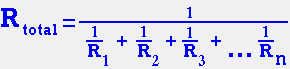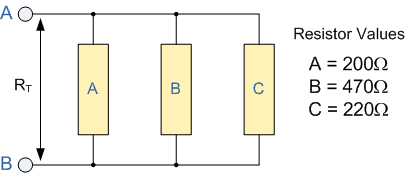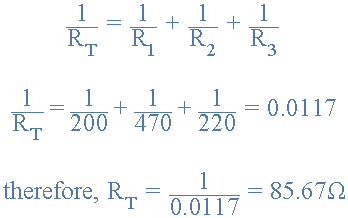# Calculating Resistors in parallel

Resistors in Parallel Calculations

Resistors are said to be connected together in "Parallel" when both of their terminals are respectively connected to each terminal of the other resistor or resistors. The voltage drop across all of the resistors in parallel is the same. "R" noted in the formula below is in OHMS.For example, find the total resistance of the following parallel resistors network:Then the total resistance RT across the terminals A and B is calculated as follow:One important point to remember about resistors in parallel, is that the total circuit resistance (RT) of any two or more resistors connected together in parallel will always be LESS than the value of the smallest resistor.